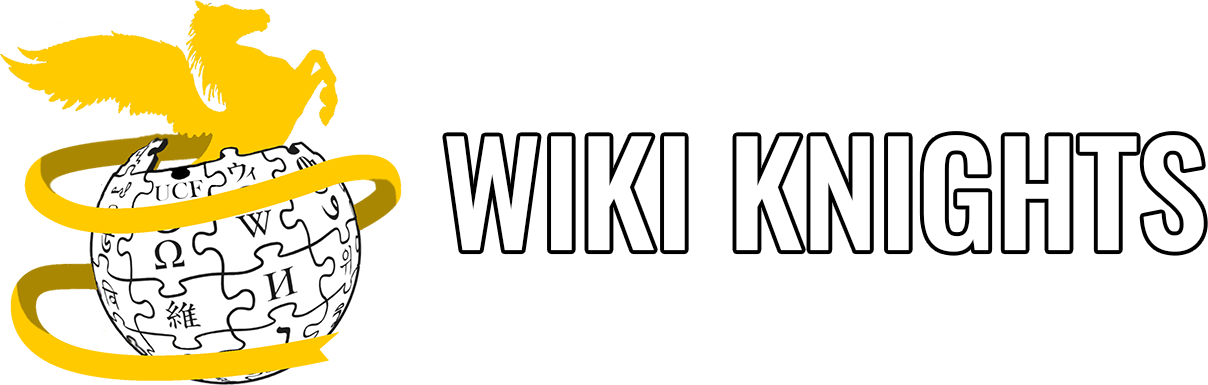# Variable Practice

Created by Jerrett Longworth in February 2022.

Here are some exercises that will help you get a better grasp of using variables! (Try solving these by hand without a compiler!)

1. Write one line of code that declares an integer called `cups_of_tea` and assigns to it a value of 5.

2. What is wrong with the following code? How can you fix it?

``````#include <stdio.h>

int main(void)
{
printf("The value of x is %d.\n", x);

int x = 7;

return 0;
}``````
1. What is wrong with the following code? How can you fix it?
``````#include <stdio.h>

int main(void)
{
ounces_of_milk = 16.0;
printf("You have %lf ounces of milk.\n", ounces_of_milk);

return 0;
}``````
1. What is the final output of this program?
``````#include <stdio.h>

int main(void)
{
int apples = 16;
int oranges = 90;

apples = oranges;
oranges = apples;

printf("You have %d apples and %d oranges.\n", apples, oranges);

return 0;
}``````